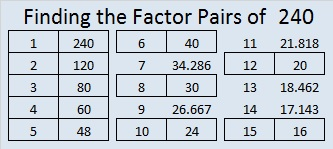# A Forest of 240 Factor Trees

• 240 is a composite number.
• Prime factorization: 240 = 2 x 2 x 2 x 2 x 3 x 5, which can be written 2⁴ x 3 x 5
• The exponents in the prime factorization are 4, 1 and 1. Adding one to each and multiplying we get (4 + 1)(1 + 1)(1 + 1) = 5 x 2 x 2 = 20. Therefore 240 has 20 factors.
• Factors of 240: 1, 2, 3, 4, 5, 6, 8, 10, 12, 15, 16, 20, 24, 30, 40, 48, 60, 80, 120, 240
• Factor pairs: 240 = 1 x 240, 2 x 120, 3 x 80, 4 x 60, 5 x 48, 6 x 40, 8 x 30, 10 x 24, 12 x 20, or 15 x 16
• Taking the factor pair with the largest square number factor, we get √240 = (√16)(√15) = 4√15 ≈ 15.4922 + 4 + 6 + 8 + 10 + 12 + 14 + 16 + 18 + 20 + 22 + 24 + 26 + 28 + 30 = 240; that’s the sum of the first 15 even numbers.

Because 240 has so many factors, it is possible to make MANY different factor trees that create a forest of 240 factor trees. This post only contains eleven of those many possibilities. The two trees below demonstrate different permutations that can be made from the same basic tree. The mirror images of both, as well as mirror images of parts of either tree, would be other permutations.A good way to make a factor tree for a composite number is to begin with one of its factor pairs and then make factor trees for the composite numbers in that factor pair.

This forest of 240 factor trees is dedicated to Joseph Nebus. Read the comments to his post, You might also like, because, I don’t know why, to discover why I was inspired to create images of parts of this forest.

1.Joseph Nebus